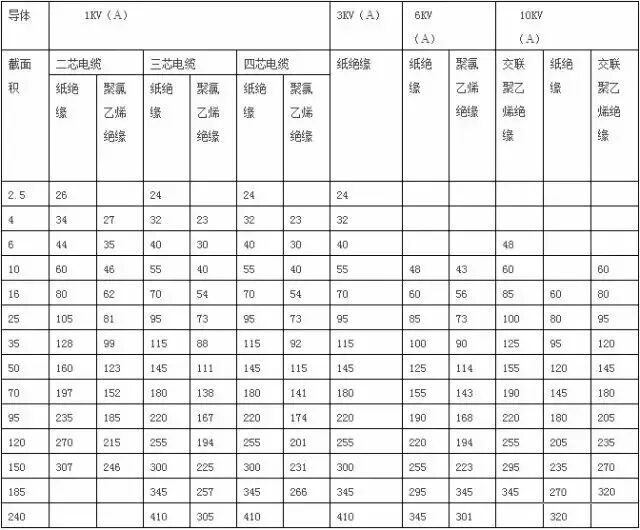一、照明电路电流计算及熔丝刀闸的选择

(取系数1.1)

QS--------刀闸

IR---------熔丝

I=P/(×U×cosΦ×n)=P/(1.73×380×0.85×0.95)=28(安)

I=P/(×U)=10/(1.73×0.38)=15.21（安）

I=S/(×U)=20/(1.73×0.38)=30.45(安)

cosΦ=有功/=1000/=1000/1044=0.957

cosΦ=有功/=1000/=1/1.22=0.81

（1）经验口诀公式：10千瓦×2=20（安）

（2）已知 U=380V  P=10千瓦  cosΦ=0.85   n=0.95

I= P/(×U×cosΦ×n)=10/(1.73×0.38×0.85×0.95)=20(安)S=P×系数×M=30×4×0.2

=24平方毫米

S——导线截面

M——员荷距（千瓦/公里）

S=4×40×0.35

S=56平方毫米，整定为70平方毫米

S=24×20×0.1

S=48平方毫米，整定为50平方毫米1、铜芯电缆的载流量为表中数值乘以系数1.3倍；

2、本表格载流量为单根电缆容量；

3、单芯塑料电缆为三角形排列，中心距等于电缆外径。1、铜芯电缆的载流量为表中数值乘以系数1.3倍；

2、本表格载流量为单根电缆容量；

3、单芯塑料电缆为三角形排列，中心距等于电缆外径

•中国PLC开发培训领导者
• 关注我们岩天PLC 岩天教育培训集团

• 电话咨询
• 在线咨询
• 在线报名
• 二维码
• 返回顶部